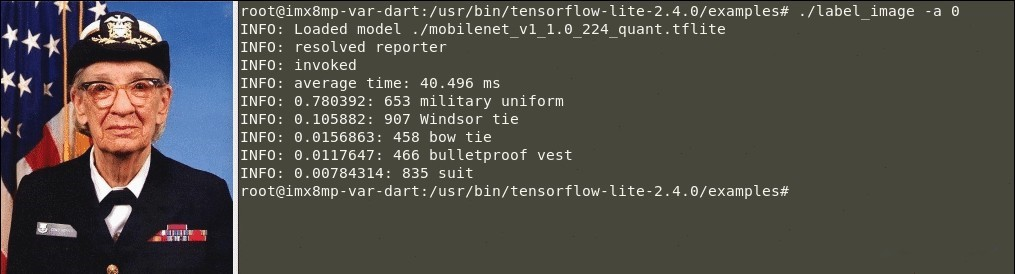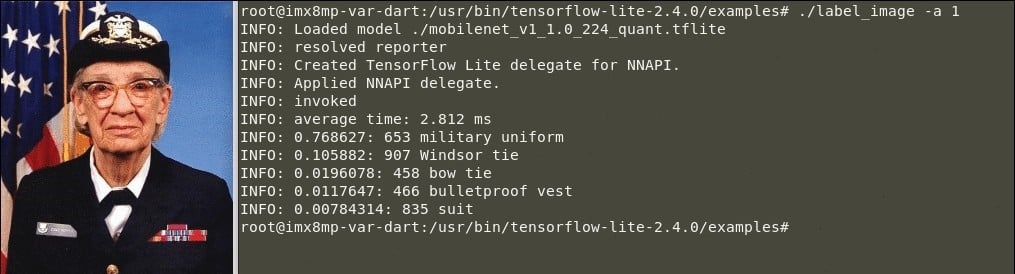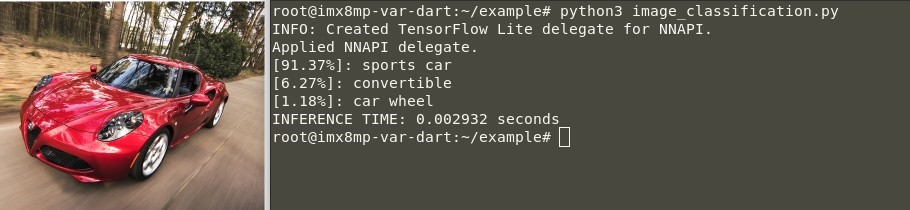# Chapter 1. Introduction

This article provides step by step instructions on how to start developing your own Machine Learning (ML) applications using Variscite’s System on Modules. It shows how to build an image using the Yocto Linux BSP with a collection of development tools, utilities, and libraries for building ML applications from the NXP eIQ™ ML Software Development Environment, how to run the default examples built with the image, and finally, how to write a simple ML example from scratch.

## 1.1. eIQ™ Machine Learning Software Development Environment Overview

eIQ™ allows users to easily develop a complete system-level application to solve ML problems related to vision, audio, time-series data and so on. Common examples are face recognition, pose estimation, gesture recognition, speech accents interpretation, etc.
The latest software stack from eIQ™ includes an ML workflow tool called eIQ™ Toolkit, which can be used to learn more about ML and to train your own ML model through the eIQ Portal; it also includes the eIQ™ Inference that enables support for inference engines, neural network compilers, and optimized libraries such as TensorFlow Lite, Arm NN, ONNX Runtime, PyTorch, OpenCV and DeepViewRT.

## 1.2. Prerequisites

### 1.2.1 Supported Variscite SoM

The DART-MX8M-PLUS and VAR-SOM-MX8M-PLUS are based on the NXP i.MX 8M Plus processor, which includes a Neural Processing Unit (NPU); a dedicated AI/ML accelerator. The NPU helps to achieve a high performance during the inference process on ML applications.

Although this article focuses on modules that are based on the i.MX 8M Plus processor, other modules based on the NXP i.MX 8 and i.MX 8M families can also be used for ML applications. In these cases, the GPU or CPU is used for the inference process instead of the NPU.

### 1.2.2. Yocto Linux BSP with eIQ™ Enablement

To build an image with the eIQ™ inference engines and libraries:

1. Follow sections 1 & 3 in the appropriate “Build Yocto from source code” guide in variwiki.com to setup your build host and retrieve the Yocto sources for the SOM and Yocto version you want to use.

``````…
\$ repo init -u https://github.com/varigit/variscite-bsp-platform.git -b <tag_name> -m <manifest_name> ①
\$ repo sync -j\$(nproc) ②
``````

❶E.g., replace <tag_name> with fsl-hardknott; <manifest_name> with imx-5.10.35-2.0.0-var01.xml
❷ The repo sync step may take a while to complete ☕

2. Prepare the environment to build the image for the chosen module:

``\$ MACHINE=<module_name> DISTRO=fsl-imx-xwayland . var-setup-release.sh -b build_xwayland ①``

❶ E.g., replace <module_name> with imx8mp-var-dart

3. Use the imx-image-full image to build the eIQ™ ML packages:

``\$ bitbake imx-image-full ①``

❶ This step may take several hours to complete depending on your computer’s specifications ☕

4. Flash the full image to the SD card:

1. The built image can be found in the following folder:

🗀 \${BUILD}/tmp/deploy/images/<module_name>

``\$ zcat imx-image-full-<module_name>.wic.gz | sudo dd of=/dev/sd<x> bs=1M status=progress conv=fsync ①``

❶E.g., replace <module_name> with imx8mp-var-dart; <x> with b

⚠️  BE CAREFUL. Use the dmesg or lsblk commands to check the correct SD card device name.

# Chapter 2. eIQ™ Machine Learning Default Applications

Due to a vast number of ML subcategories, this article only describes an example from the supervised learning subcategory, which is the image classification problem. To learn how the classification problem works, we will run an example from eIQ™ that uses a trained starter model provided by TensorFlow, and the inference engine from TensorFlow Lite built along with the Yocto Linux BSP.

## 2.1.   TensorFlow Lite

The most popular and well-supported inference engine and library from eIQ™ is TensorFlow Lite developed by Google. While TensorFlow is a popular open-source platform for Machine Learning that can be used for both network training and inference, TensorFlow Lite is a set of tools specifically designed to convert and run inference from TensorFlow models on embedded devices with lower latency and smaller binary size.

### 2.1.1 Image Classification Overview

Image classification is a classification problem from the supervised learning subcategory, which can be used to identify what an image represents without relying on hard-coded rules. To make this work, we need to train an image classification model to recognize various classes of images. For example, we can train a model to recognize many objects, such as vehicles, people, traffic lights, types of fruits or animals and so on.

To train an image classification model, the training must be fed with images and their associated labels. It requires having hundreds or thousands of images per label so the model can efficiently learn to predict whether new images belong to any of the classes it has been trained on. The process of the model making a prediction on a new input image is called inference.

The training process of a new model takes a lot of time, depending on several aspects such as how the neural network is defined and the amount of data that is used to train the model.

The model used in this article was previously trained and tested by TensorFlow. As mentioned in the first chapter of this article, you can also create, optimize, debug, convert and export your own ML model using the NXP eIQ™ Portal.

When we provide a new image as data input to the model, it will output the probability of the image representing each of the objects it was trained on. An output example may be as follows in Table 1:

Table 1. Probability Results Example

 Object Name (Label) Probability Dog 0.91 Cat 0.07 Rabbit 0.02

The image classification model comes with the corresponding labels file, which contains the list of objects the model was trained on (for example, see tensorflow/lite/java/ovic/src/testdata/labels.txt in the following example source code), and each number in the output corresponds to a label in the labels file. In the above example, associating the output with the three labels the model was trained on, it shows a high probability that the image in this case represents a dog.

### 2.1.2. Image Classification Example

The full image built with the eIQ™ packages provides an image classification example written in C++, and a similar one written in Python. The C++ API from TensorFlow provides an option to choose the compute unit to run the inference, whereas the Python bindings does not provide this option, so the examples that are written in Python only run inference on the NPU.

This section explains how to run the default example written in C++. The next section explains how to run the default example written in Phyton, where you will learn how to write an example from scratch using the Python bindings from TensorFlow Lite.
The C++ example already comes with a trained starter model, labels file and an image example to be used as an input to the inference process – see table 2 below:

Table 2. Image Classification Example Details

 Example Name Language Default Model Default Labels Default Input label_image C++/Python mobilenet_v1_1.0_224 _quant.tflite labels.txt grace_hopper.bmp

• Boot the board and go to the following folder where the image classification example is located:

``\$ cd /usr/bin/tensorflow-lite-<version>/examples ①``

❶ E.g., replace <version> with 2.4.1

• Use the following arguments to use different model / labels / image input data files:

``\$ ./label_image -m <model_file_name.tflite> -l <labels_file_name.txt> -i <image_file_name.extension>``

If no argument is specified, the example uses the default arguments from Table 2.

Example in C++ (CPU)

1.Execute the label image example using the “-a” argument with a “0” value to run the inference on the CPU:

``\$ ./label_image -a 0``
1. The output of a successful classification should be similar to the following:Figure 1. Running TensorFlow Lite Image Classification Example (CPU Inference)

🕒 Inference Time on CPU: 40.496 milliseconds.

Example in C++ (NPU)

1.Execute the label image example using the “-a” argument with a “1” value to run the inference on the NPU:

``\$ ./label_image -a 1``
1. The output of a successful classification should be similar to the following:Figure 2. Running TensorFlow Lite Image Classification Example (NPU Inference)

🕒 Inference Time on NPU: 2.812 milliseconds.

This message indicates that the inference is running on NPU:

“INFO: Applied NNAPI delegate.”

The source code for the label_image example is available at the tensorflow-imx repository:

# Chapter 3. eIQ™ ML Application Development

This section is a step-by-step guide for developing a simple image classification example using a starter model, a labels file and an image as data input. This example is written in Python using the TensorFlow Lite Python API.

The steps below show how to open and resize the image according to the input size of the model, how to load the image as input data through the inference process on the NPU, and finally how to analyze the output to get the probability results.

The source code for this image classification example is available at:

In the above repository, you can find additional examples, such as:
Image file, video file and real-time video stream classification.
Image file, video file and real-time video stream detection (which returns the classification label along with the object’s position in the image, and draws a rectangle around it).
User interface application, etc.

## 3.1.   Image Classification from Scratch

### 3.1.1. Get Started

1. Create a directory and retrieve the image classification starter model and a free image to be used as data input:

``````\$ mkdir ~/example && cd ~/example
\$ wget https://raw.githubusercontent.com/varigit/var-demos/master/machine-learning-demos/tflite/classification/media/image.jpg
``````

This image is just an example, feel free to use any other image.

a. Extract the model in the directory:

``\$ unzip mobilenet_v1_1.0_224_quant_and_labels.zip``
1. Remove the redundant files:
``\$ rm -rf __MACOSX/ mobilenet_v1_1.0_224_quant_and_labels.zip``

2. Create the .py file to start writing the source code:

``\$ touch ~/example/image_classification.py``

3. The folder structure should look like this:

``````.
├── image_classification.py
├── image.jpg
├── labels_mobilenet_quant_v1_224.txt
└── mobilenet_v1_1.0_224_quant.tflite

0 directories, 4 files``````

### 3.1.2. Edit image_classification.py and Write the Source Code

1. First, import the Time, NumPy, Pillow, and the TensorFlow Lite libraries:

``````1 from time import time
2
3 import numpy as np
4 from PIL import Image
5 from tflite_runtime.interpreter import Interpreter``````

2. Open and read the lines from the labels file of the classification model:

``````6 with open('labels_mobilenet_quant_v1_224.txt') as f:

3. Use the Interpreter module to load the image classification model and allocate its tensors:

``````8 interpreter = tf.Interpreter(model_path="mobilenet_v1_1.0_224_quant.tflite")
9 interpreter.allocate_tensors()``````

4. Get the details from the input and output tensors of the image classification model:

``````10 input_details = interpreter.get_input_details()
11 output_details = interpreter.get_output_details()``````

5. Open the image and resize it according to the input size of the model:

``````12 with Image.open("image.jpg") as im:
13     _, height, width, _ = input_details['shape']
14     image = im.resize((width, height))
15     image = np.expand_dims(image, axis=0)``````

6. To load the resized image as data input to the NPU set the input tensor:

``16 interpreter.set_tensor(input_details['index'], image)``

7. Call the invoke method to start the inference on the NPU:

``````17 interpreter.invoke() ①
18
19 start = time()
20 interpreter.invoke() ②
21 final = time()``````

❶ The first call of the invoke method takes longer than usual due to initialization steps;
❷ To get the actual time the NPU takes to run the inference, call the invoke method again.

The initialization steps are also called the warm-up phase. They are only needed once, at the beginning of the application.

8. Get the output details after running the inference on the NPU:

``22 output_details = interpreter.get_output_details()``

9. Get the three most relevant probabilities as explained in the 2.1.1 Image Classification Overview section:

``````23 output = np.squeeze(interpreter.get_tensor(output_details['index']))
25 results = output.argsort()[-3:][::-1]``````

10. Print the labels and their probabilities:

``````26 for i in results:
27     score = float(output[i] / 255.0)
28     print("[{:.2%}]: {}".format(score, labels[i]))
29``````

11. And finally, print the inference time:

``30 print("INFERENCE TIME: {:.6f} seconds".format(final-start))``

### 3.1.3 Test the Example on the Chosen Module

1. Copy the example folder to the target board:

``\$ scp -r ~/example root@<target-ip>:/home/root``
1. On the board, execute the following commands:
``````# cd /home/root/example
# python3 image_classification.py``````
1. The output of a successful classification should be similar to the following:Figure 3. TensorFlow Lite Image Classification Example Input (NPU Inference)

🕒 Inference Time on NPU: 2.9 milliseconds.

As you can see, the model has predicted a high probability (91.37%) that the image represents a sports car.

This example can be further used as a base for any application related to image classification problems. For instance, you can modify the source code to use a video file or a live video stream from a camera, instead of an image file input, using OpenCV and GStreamer, as done in the var- demos repository mentioned above.